# Dannica Corporation produces products that it sells for \$40 each. Variable costs per unit are \$25,...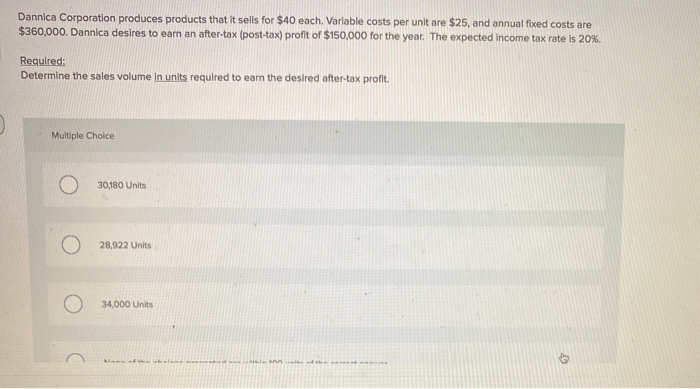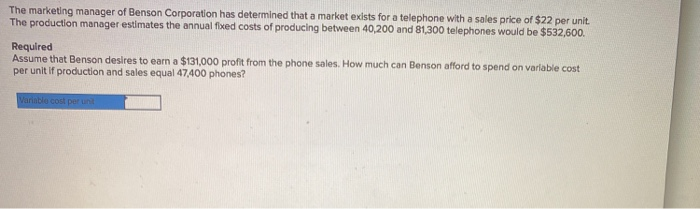Dannica Corporation produces products that it sells for \$40 each. Variable costs per unit are \$25, and annual fixed costs are \$360,000. Dannica desires to earn an after-tax (post-tax) profit of \$150,000 for the year. The expected income tax rate is 20%. Required: Determine the sales volume in units required to earn the desired after-tax profit. Multiple Choice 30,180 Units 28,922 Units 34,000 Units NR NA
The marketing manager of Benson Corporation has determined that a market exists for a telephone with a sales price of \$22 per unit . The production manager estimates the annual fixed costs of producing between 40,200 and 81300 telephones would be \$532,600. Required Assume that Benson desires to earn a \$131,000 profit from the phone sales. How much can Benson afford to spend on variable cost per unit if production and sales equal 47,400 phones? Variable cost per un

1. units required to earn a desired profit = fixed cost + desired amount of profit

contribution per unit

contribution per unit is not given, therefore,

contribution = selling price - variable cost per unit

= 40-25 = 15

units required = 360,000+150,000 / 15

= 510,000/15

= 34,000 units.

2. as per the question,

units= 47,400 fixed cost = 532600, (because the units produced is between 40,200 and 81,300.)

desired profit = 131,000 variable cost = as it is not given, assume as V

price = 22

we know that,

units required to earn desired profit = fixed cost + desired profit / contribution per unit

contribution = selling price - variable cost

= 22 - V.

substituting in above formula gives,

47,400 = 532,600 + 131,000 /22-V

47,400 = 663,600 / 22-V

47,400 (22- V) = 663,600

22-V = 663,600 / 47400

22-V = 14

V = 22-14

Variable cost = 8.

##### Add Answer of: Dannica Corporation produces products that it sells for \$40 each. Variable costs per unit are \$25,...
Similar Homework Help Questions
• ### Finch Corporation produces products that it sells for \$21 each. Variable costs per unit are \$4,...

Finch Corporation produces products that it sells for \$21 each. Variable costs per unit are \$4, and annual fixed costs are \$341,700. Finch desires to earn a profit of \$79,900. Required Use the equation method to determine the break-even point in units and dollars. Determine the sales volume in units and dollars required to earn the desired profit.

• ### Franklin Corporation produces products that it sells for \$18 each. Variable costs per unit are \$6,...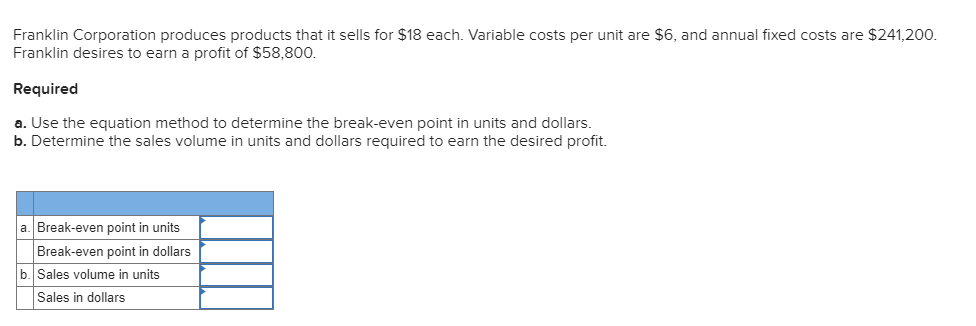Franklin Corporation produces products that it sells for \$18 each. Variable costs per unit are \$6, and annual fixed costs are \$241,200. Franklin desires to earn a profit of \$58,800. Required a. Use the equation method to determine the break-even point in units and dollars. b. Determine the sales volume in units and dollars required to earn the desired profit. a Break-even point in units Break-even point in dollars b. Sales volume in units Sales in dollars

• ### Baird Corporation produces products that it sells for \$21 each. Variable costs per unit are \$6,...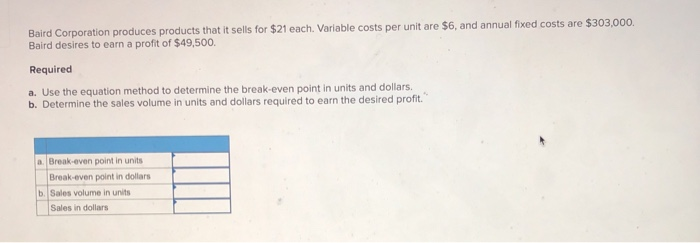Baird Corporation produces products that it sells for \$21 each. Variable costs per unit are \$6, and annual fixed costs are \$303,000. Baird desires to earn a profit of \$49,500. Required a. Use the equation method to determine the break-even point in units and dollars. b. Determine the sales volume in units and dollars required to earn the desired profit." a Break-even point in units Break-even point in dollars b. Sales volume in units Sales in dollars

• ### Vernon Corporation produces products that it sells for \$19 each. Variable costs per unit are \$9,...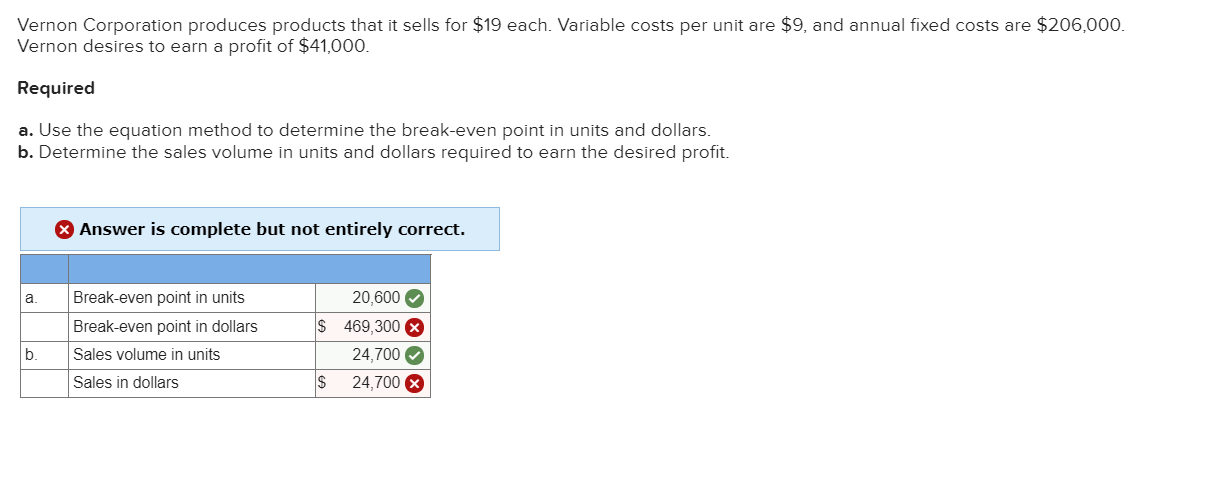Vernon Corporation produces products that it sells for \$19 each. Variable costs per unit are \$9, and annual fixed costs are \$206,000. Vernon desires to earn a profit of \$41,000. Required a. Use the equation method to determine the break-even point in units and dollars. b. Determine the sales volume in units and dollars required to earn the desired profit. Answer is complete but not entirely correct. a. Break-even point in units Break-even point in dollars Sales volume in units...

• ### Q.1 Rooney Corporation produces products that it sells for \$18 each. Variable costs per unit are...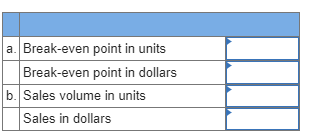Q.1 Rooney Corporation produces products that it sells for \$18 each. Variable costs per unit are \$9, and annual fixed costs are \$189,900. Rooney desires to earn a profit of \$33,300. Required Use the equation method to determine the break-even point in units and dollars. Determine the sales volume in units and dollars required to earn the desired profit. a. Break-even point in units Break-even point in dollars b. Sales volume in units Sales in dollars

• ### The marketing manager of Jordan Corporation has determined that a market exists for a telephone with...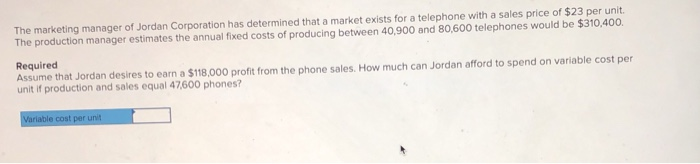The marketing manager of Jordan Corporation has determined that a market exists for a telephone with a sales price of \$23 per unit. The production manager estimates the annual fixed costs of producing between 40,900 and 80,600 telephones would be \$310,400. Required Assume that Jordan desires to earn a \$118,000 profit from the phone sales. How much can Jordan afford to spend on variable cost per unit if production and sales equal 47,600 phones? Variable cost per unit

• ### The marketing manager of Finch Corporation has determined that a market exists for a telephone with...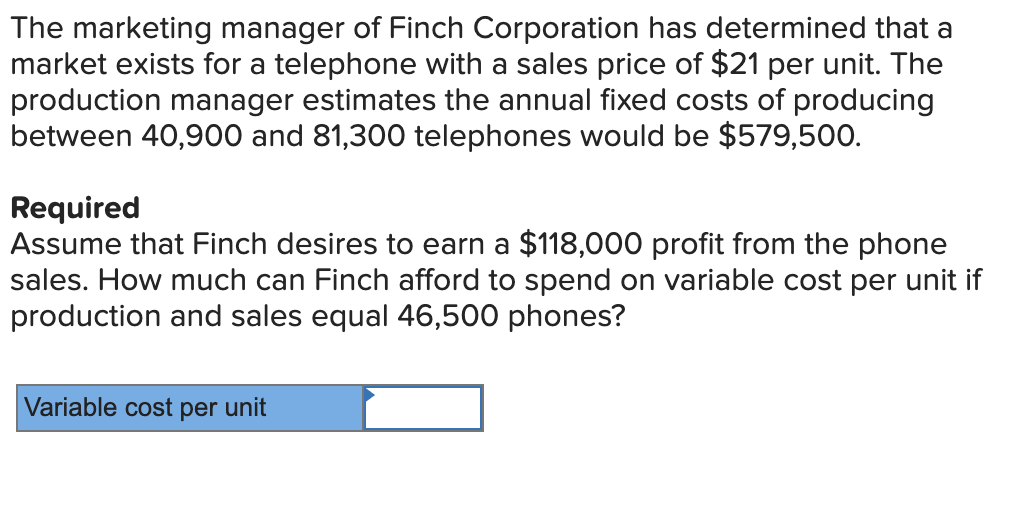The marketing manager of Finch Corporation has determined that a market exists for a telephone with a sales price of \$21 per unit. The production manager estimates the annual fixed costs of producing between 40,900 and 81,300 telephones would be \$579,500. Required Assume that Finch desires to earn a \$118,000 profit from the phone sales. How much can Finch afford to spend on variable cost per unit if production and sales equal 46,500 phones? Variable cost per unit

• ### The marketing manager of Thornton Corporation has determined that a market exists for a telephone with...

The marketing manager of Thornton Corporation has determined that a market exists for a telephone with a sales price of \$20 per unit. The production manager estimates the annual fixed costs of producing between 41,900 and 80,400 telephones would be \$196,800. Required: Assume that Thornton desires to earn a \$128,000 profit from the phone sales. How much can Thornton afford to spend on variable cost per unit if production and sales equal 46,400 phones?

• ### Benson Company makes and sells products with variable costs of \$41 each. Benson incurs annual fixed...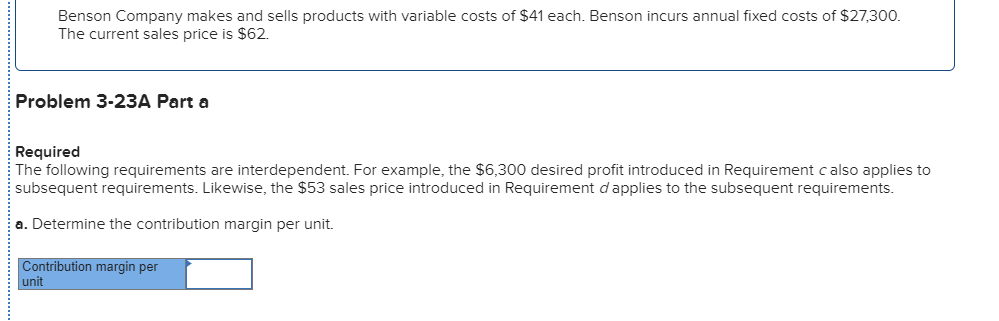Benson Company makes and sells products with variable costs of \$41 each. Benson incurs annual fixed costs of \$27,300. The current sales price is \$62. Problem 3-23A Part a Required The following requirements are interdependent. For example, the \$6,300 desired profit introduced in Requirement calso applies to subsequent requirements. Likewise, the \$53 sales price introduced in Requirement d applies to the subsequent requirements. a. Determine the contribution margin per unit. Contribution margin per unit b. Determine the break-even point in...

• ### Fowler Company produces a product that sells for \$200 per unit and has a variable cost...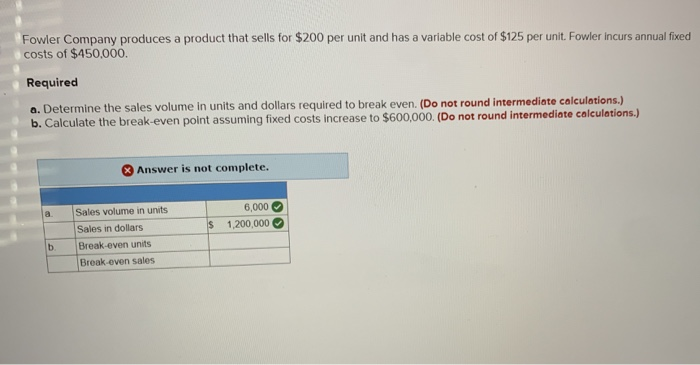Fowler Company produces a product that sells for \$200 per unit and has a variable cost of \$125 per unit. Fowler incurs annual fixed costs of \$450,000 Required a. Determine the sales volume in units and dollars required to break even. (Do not round intermediate calculations.) b. Calculate the break-even point assuming fixed costs increase to \$600,000. (Do not round intermediate calculations.) Answer is not complete. 6,000 \$ 1,200,000 Sales volume in units Sales in dollars Break-even units Break-even sales...

Free Homework App